# Full Factorial DOE with JMP

### Full Factorial DOE

In a full factorial experiment, all of the possible combinations of factors and levels are created and tested. For example, for two-level design (i.e.each factor has two levels) with k factors, there are 2k possible scenarios or treatments.

1. Two factors, each with two levels, we have 22 = 4 treatments
2. Three factors, each with two levels, we have 23 = 8 treatments
3. k factors, each with two levels, we have 2k treatments

### 2k Full Factorial DOE

Full factorial DOE is used to discover the cause-and-effect relationship between the response and both individual factors and the interaction of factors. Generate an equation to describe the relationship between Y and the important Xs: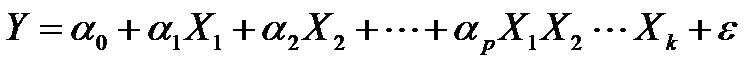Where:

• Y is the response and X1, X2 . . . Xk are the factors
• α0 is the intercept and α1, α2 . . . αp are the coefficients of the factors and interactions
• ε is the error of the model

### Two-Level Two-Factor Full Factorial

Below is a design pattern of a two-level two-factor full factorial experiment.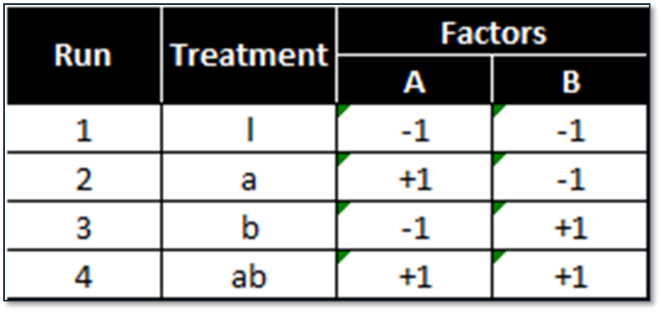2 (level) raised to 2 (factors) = 4 treatment combinations.

### Two-Level Three-Factor Full Factorial

Below is a design pattern of a two-level three-factor full factorial experiment.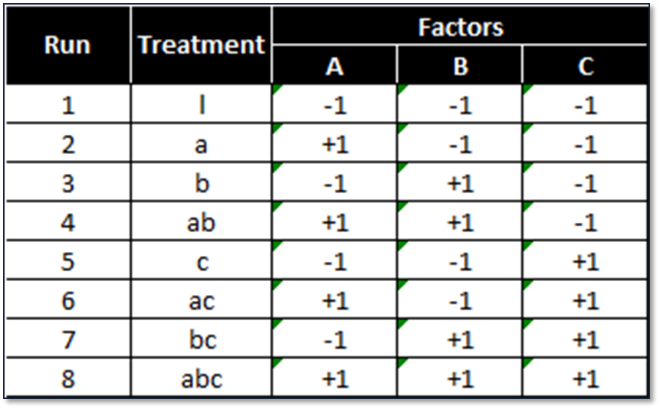2 (levels) raised to 3 (factors) = 8 treatment combinations.

### Two-Level Four-Factor Full Factorial

Below is a design pattern of a two-level four-factor full factorial experiment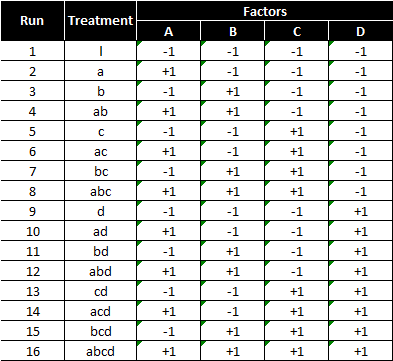2 (levels) raised to 4 (factors) = 16 treatment combinations

### Two-Level Five-Factor Full Factorial

Below is a design pattern of a two-level five-factor full factorial experiment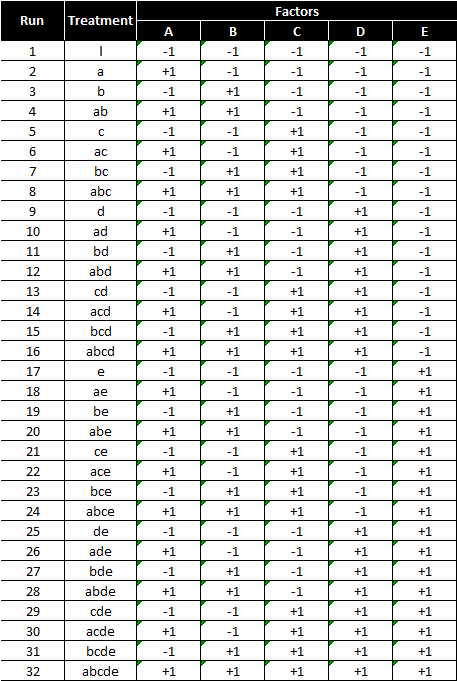2 (levels) raised to 5 (factors) = 32 treatment combinations

### Order to Run Experiments

The four design patterns shown earlier are listed in the standard order. Standard order is used to design the combinations/treatments before experiments start. When actually running the experiments, randomizing the standard order is recommended to minimize the noise.

### Replication in Experiments

Each treatment can be tested multiple times in an experiment in order to increase the degrees of freedom and improve the capability of analysis. We call this method replication.
Replicates are the number of repetitions of running an individual treatment, which increase the power of the experimental responses. The order to run the treatments in an experiment should be randomized to minimize the noise.
Advantages of replication include: helps to better identify the true sources of variation, helps estimate the true impacts of the factors on the response, and overall improves the reliability and validity of the experimental results.

### 22 Full Factorial DOE

Case study: We are running a 22 full factorial DOE to discover the cause-and-effect relationship between the cake tastiness and two factors: temperature of the oven and time length of baking. Each factor has two levels and there are four treatments in total.
We decide to run each treatment twice so that we have enough degrees of freedom to measure the impact of two factors and the interaction between two factors. Therefore, there are eight observations in response eventually.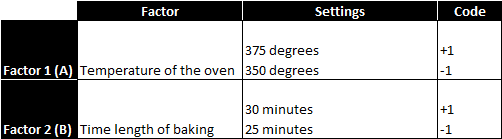The objective is to understand the main effects and the interactions of these factors on the response variable. After running the four treatments twice in a random order, we obtain the following results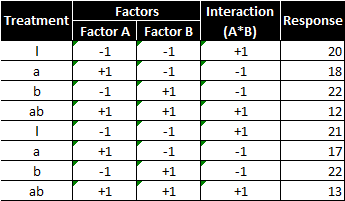There are two factors and two levels, so there would be 2^2 = 4 treatment combinations. With replicates, each treatment combination is repeated once; therefore, there are in total 8 runs in this experiment. The experiment results are consolidated into the following table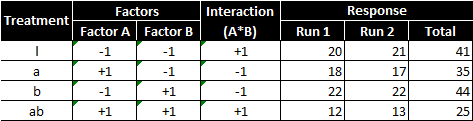The main effect of factor A is computed by averaging the difference between combinations where A was at its high settings and where A was at its low settings.
Main effect of factor A (temperature of the oven):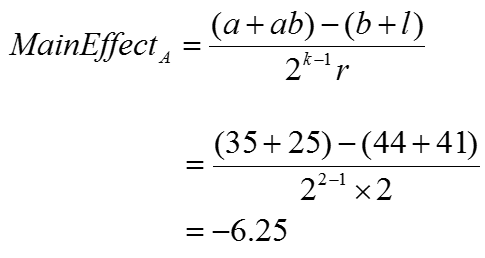Where:

• k is the number of factors
• r is the number of times individual treatments are being run

Using the formula provided, the main effect of increasing the temperature of the oven is to decrease tastiness of the cake by −6.25.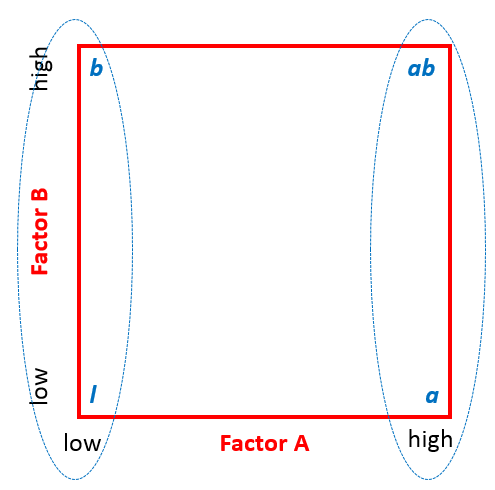The main effect of factor B, similar to A, is computed by averaging the difference between combinations where B was at its high settings and where B was at its low settings.
Main effect of factor B (time length of baking):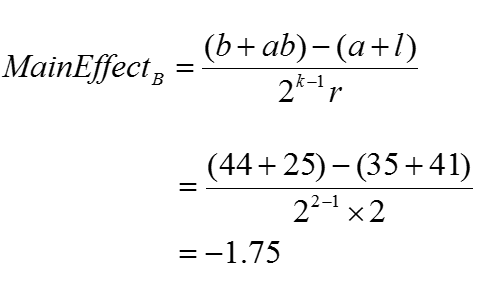Where:

• k is the number of factors
• r is the number of times individual treatments are being run

Using the formula provided, the main effect of increasing the baking time is to decrease the tastiness of the cake by −1.75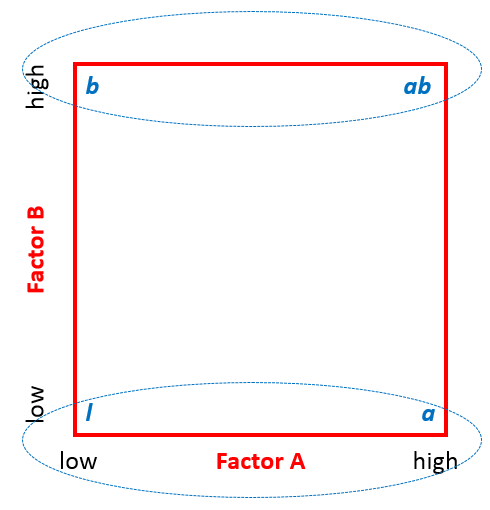The interaction effect is computed by averaging the difference between combinations where A and B were at opposite settings (low and high).

#### Interaction (i.e. A*B) effect: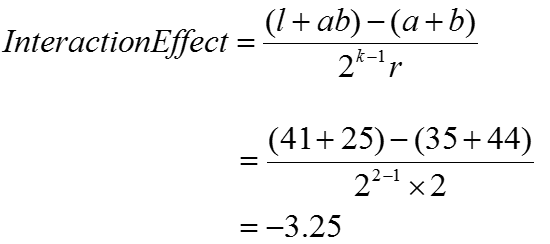Where:

• k is the number of factors
• r is the number of times individual treatments are being run

Using the formula provided, the interaction effect of the temperature and time variables on tastiness was −3.25.

####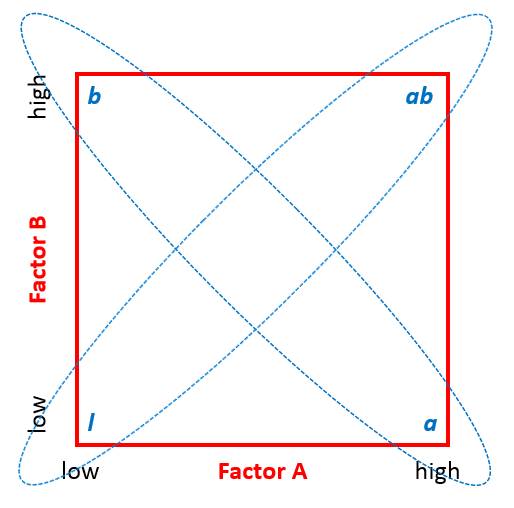Sum of squares of factors and interaction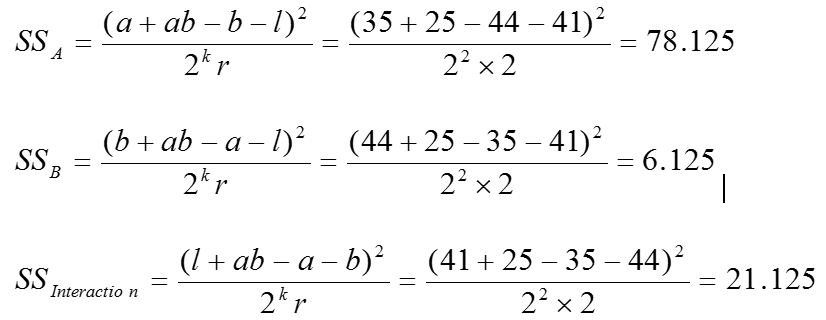Where:

• k is the number of factors
• r is the number of times individual treatments are being run

The sum of squares tells us the relative strength of each main effect and interaction. A has the strongest effect as indicated by the high SS value. The degrees of freedom are necessary to determine the mean squares value.

#### Degrees of freedom of factors and interaction: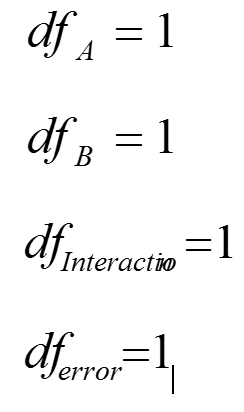Four degrees of freedom are necessary because there are three effects we are looking to understand: factor A, factor B, and the interaction between them.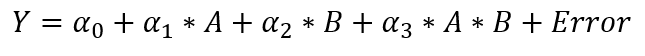#### Mean squares of factors and interaction: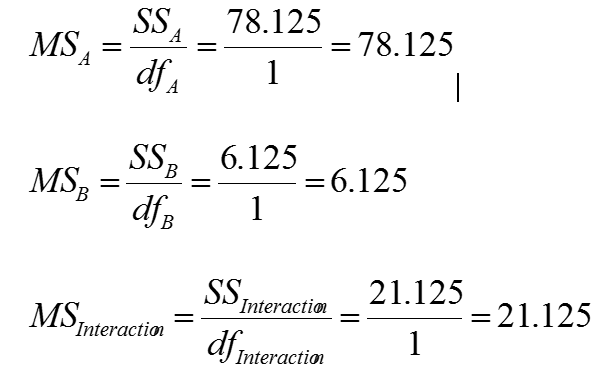### Use JMP to Run a 2k Full Factorial DOE

Step 1: Initiate the experiment design

1. Click DOE -> Custom Design
2. A window named “DOE – Custom Design” pops up
3. Double-click the name box and enter “Tastiness” into the “Response Name” box.
4. Because the tastiness metric indicates how delicious the cake is, we want to maximize it.
5. Click on the button “Add Factor”
6. Click Categorical-> 2 level
7. Enter the factor name “Temp” in the name box
8. Enter the two levels’ codes “Low” and “High” in the values boxes
9. Repeat the same steps for the second factor “Time” with two levels “Short” and “Long”
10. Click Continue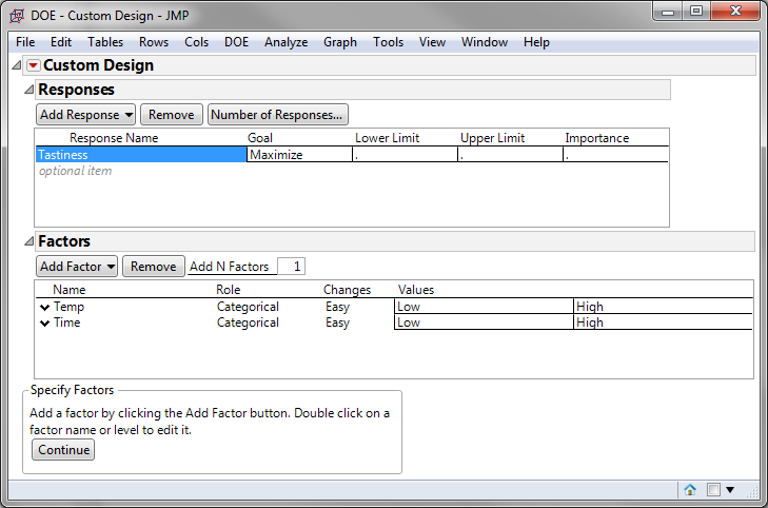Step 2: Select interaction between the two factors.

1. Click on the drop-down box of “Interactions”
2. Select “2nd”
3. The interaction variable “Temp* Time” appears in the list of factors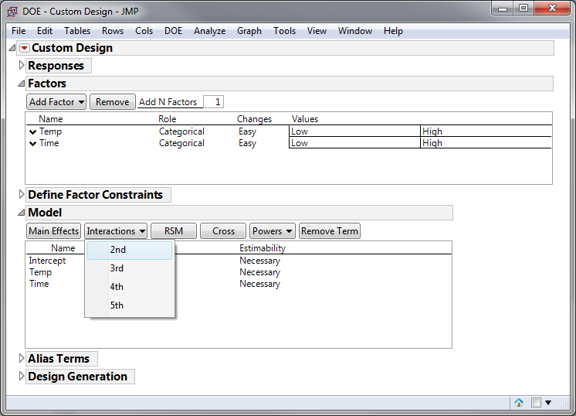Step 3: Make the design and make the table

1. Click on the “Make Design” button
2. The design pattern of the experiment appears
3. Keep “Randomize” in the “Run Order” box
4. Click on “Make Table” button
5. A table with pre-populated treatments in random run order is generated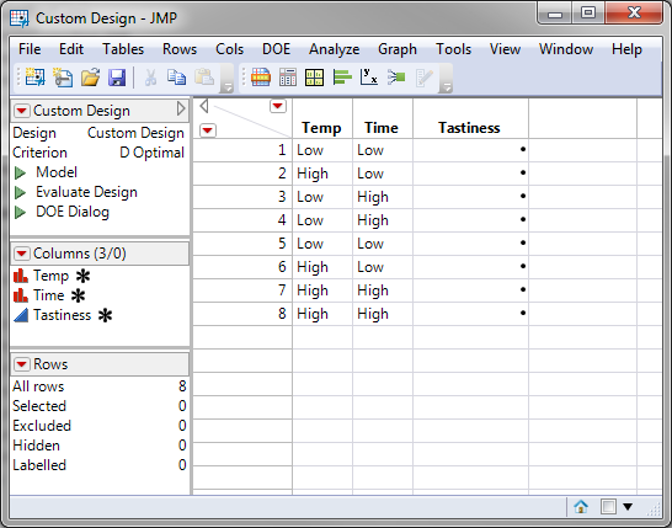Step 4: Run the experiment and record the responses in the table created by JMP.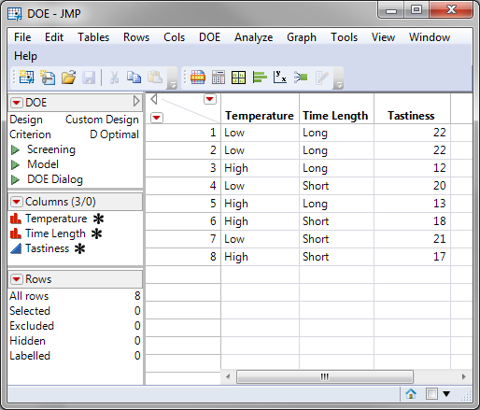Step 5: Analyze the experiment results

1. Click Analyze -> Fit Model
2. A box named “Fit Model” pops up in which the response, the two factors and their interaction term are all pre-populated into the “Y” and “Construct Model Effects” boxes respectively
3. Click on “Run” button
4. The statistics of the model appear
5. Analyze the model results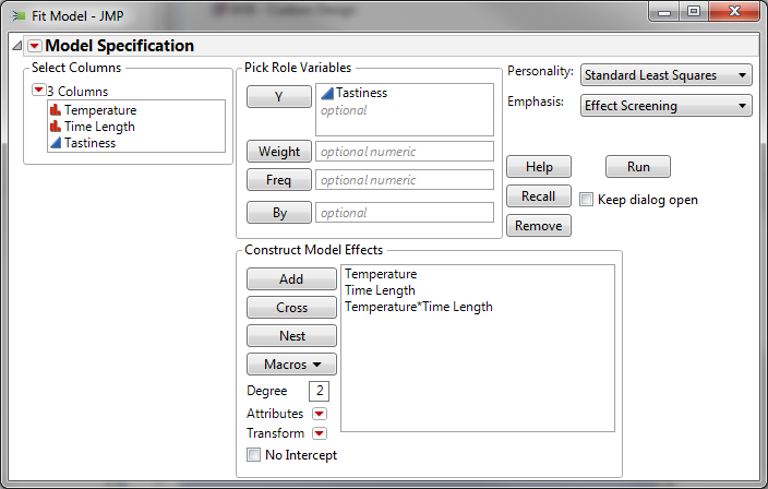Step 6: Analyze the experiment results

1. Click the red triangle next to “Response Tastiness”
2. Select “Regression Reports”
3. Select “Summary of Fit”
4. Select “Analysis of Variance”
5. Now your “Summary of Fit” and “Analysis of Variance” tables appear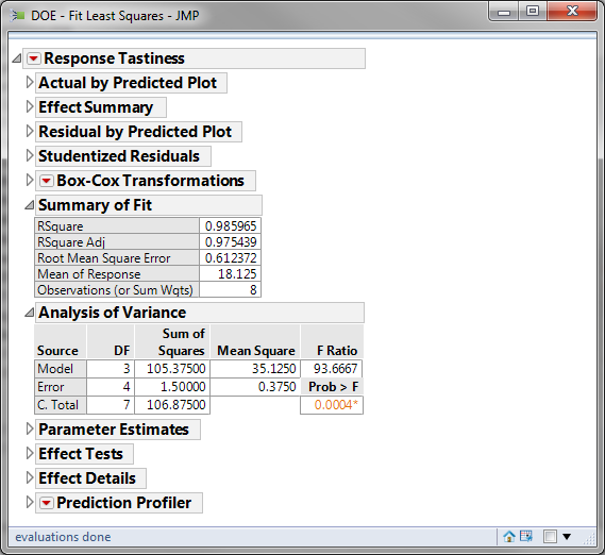High R2 value shows around 98% of the variation in the response can be explained by the model. A p-value smaller than the alpha level (0.05) indicates that the model is statistically significant (e.g. at least one of the independent variables in the model has a coefficient statistically different from zero).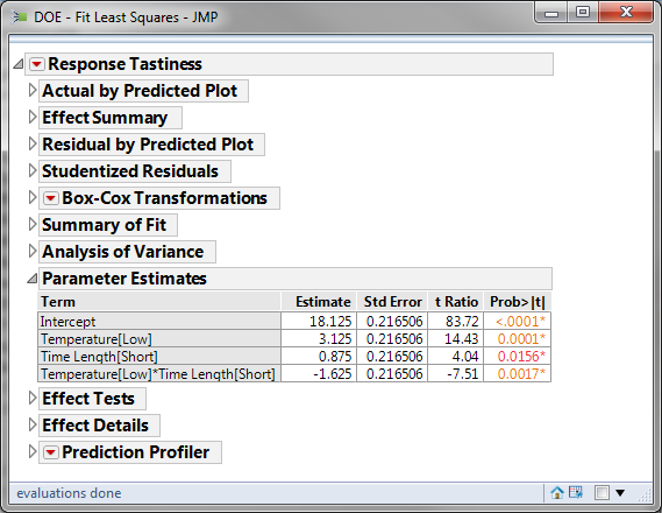Model summary: The Parameter Estimates table shows the intercept and coefficients of independent variables in the model. Since p values of all the independent variables in the mode are smaller than the alpha level (0.05), both factors and their interaction have a statistically significant impact on the response. The output provides intercept values and coefficients for a regression model equation.